Function Repository Resource:

# CycleLengthCountList

List the possible cycle length counts of permutations of a given length

Contributed by: Wolfram Staff (original content by Sriram V. Pemmaraju and Steven S. Skiena)
 ResourceFunction["CycleLengthCountList"][n] returns a list of all possible cycle length counts that represent a partitioning of permutations of size n into disjoint cycles.

## Details and Options

ResourceFunction["CycleLengthCountList"] returns a list of SparseArray objects representing {{λ11,,λ1n},,{λ{XMLElement[span, {class -> stylebox}, {XMLElement[i, {class -> ti}, {k}], 1}]},,λkn}}, where {λ{XMLElement[span, {class -> stylebox}, {XMLElement[i, {class -> ti}, {i}], 1}]},,λin} is a list of possible cycle length counts for an n-permutation.
The list {λ{XMLElement[span, {class -> stylebox}, {XMLElement[i, {class -> ti}, {i}], 1}]},,λin} is known as a cycle type.

## Examples

### Basic Examples (2)

Possible cycle length counts for 5-permutations:

 In:=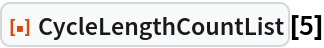Out=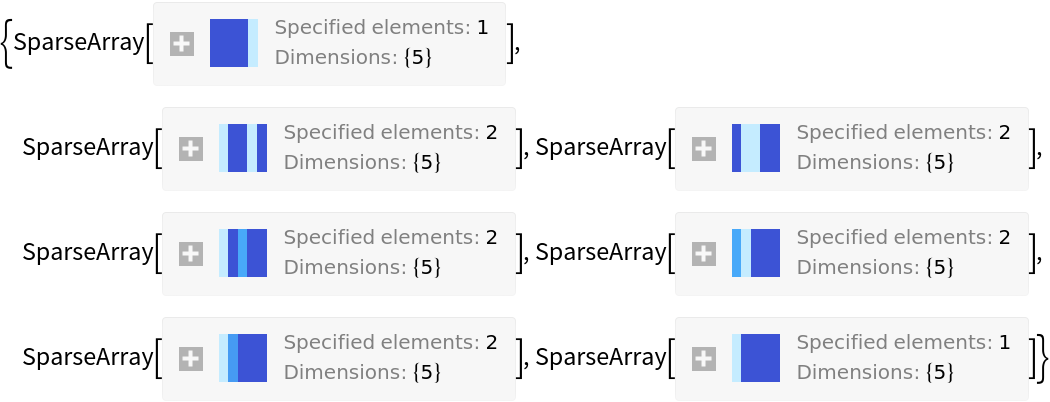See the values:

 In:=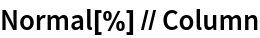Out=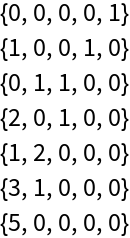### Properties and Relations (3)

There are exactly as many cycle types of n-permutations as there are integer partitions of n:

 In:=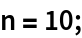In:=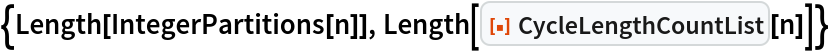Out=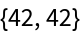Use the resource function PermutationCountByCycleLength to count the number of permutations of each possible type:

 In:=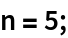In:=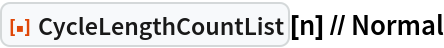Out=In:=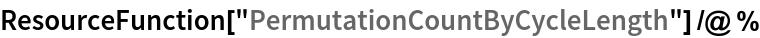Out=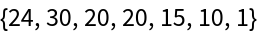As expected, there are n! permutations in total:

 In:=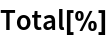Out=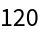In:=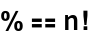Out=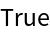Counting possible permutations of each type is the same as tallying cycle length counts in the Permutations list:

 In:=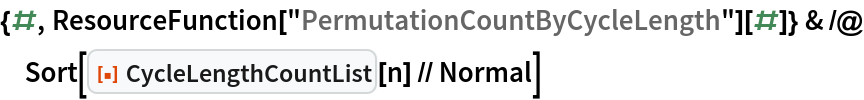Out=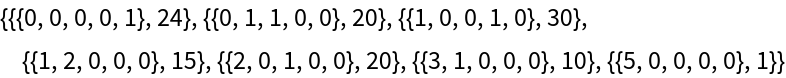In:=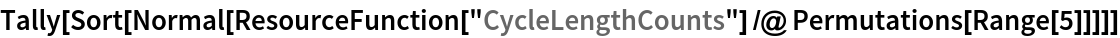Out=In:=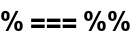Out=## Version History

• 1.0.0 – 09 July 2020

## Author Notes

Watson's book and the DLMF associate two functions with Lommel, denoted sμ,ν(z) and Sμ,ν(z). LommelS implements the latter one, since the former is undefined when μ±ν is an odd negative integer.